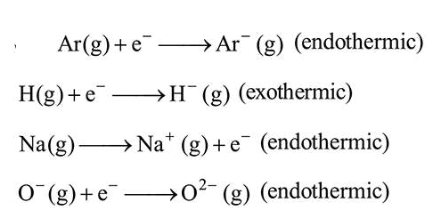# The process that is NOT endothermic in nature is :Question:

The process that is NOT endothermic in nature is :

1. $\operatorname{Ar}(g)+e^{-} \rightarrow \operatorname{Ar}^{-}(g)$

2. $\mathrm{H}(\mathrm{g})+\mathrm{e}^{-} \rightarrow \mathrm{H}^{-}(\mathrm{g})$

3. $\mathrm{O}^{-}(\mathrm{g})+\mathrm{e}^{-} \rightarrow \mathrm{O}^{2-}(\mathrm{g})$

4. $\mathrm{Na}(\mathrm{g}) \rightarrow \mathrm{Na}^{+}(\mathrm{g})+\mathrm{e}^{-}$

Correct Option: , 2

Solution:- Electron gaining enthalpy (EGE) of $\mathrm{H}(\mathrm{g})$ is negative while that of $\operatorname{Ar}(\mathrm{g})$ is positive due to $n s^{2} n p^{6}$ configuration.

- Second EGE is always positive for an atom.

- Ionization potential of an atom is positive.